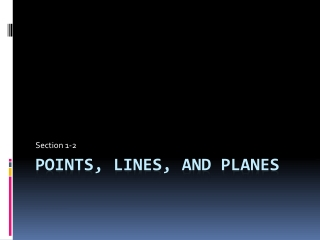DownloadDownload PresentationPoints, Lines, and Planes

# Points, Lines, and Planes

Télécharger la présentation## Points, Lines, and Planes

- - - - - - - - - - - - - - - - - - - - - - - - - - - E N D - - - - - - - - - - - - - - - - - - - - - - - - - - -
##### Presentation Transcript

1. Section 1-2 Points, Lines, and Planes

2. Introduction • This first chapter will give you the necessary definitions to understand geometry • Please remember the conventions given as that will be how you are expected to present a final answer • Do what you can to remember these definitions • That was a hint

3. Definitions • Point • A location • Has no size and takes up no space • Shown as a dot or a corner • Usually labeled with a letter • Naming convention • Read as “point A” • Written as ∙A • Partner Up • Give some real life examples of points

4. Definitions • Line • Straight connector between two points • Has no thickness • Extends infinitely in both directions • Labeled with at least two points or an italicized, lowercase letter at one end m F E

5. Definitions • Naming conventions (previous examples) • If given points • Read as “line EF” or “line FE” • Written as or • If given the letter at the end • Written as it’s read; “line m”

6. Definitions D E • Plane • A surface • Has no thickness • Usually shown as a shaded shape • The most difficult of the three definitions • Naming Conventions • If given a group of points NOT on the same line • Read and written as “plane DEF” • If given a capital letter off by itself • Read and written as “plane P” • Partner Up • Come up with 4 examples of planes F P

7. Other Definitions • Collinear points • Two or more points on the same line • Coplanar • Two or more points or lines on the same plane

8. Example T • What are other ways to name line QT? • What are other ways to name plane P? • Name a set of three collinear points. • Name a set of four coplanar points. m R Q V S P W n

9. More Definitions • Line Segment • A measurable piece of a line • Named by it two endpoints, but sometimes by a single capital letter • Naming Conventions • Read “segment AB” • Written as or B A

10. More Definitions • Ray • Half a line • One end is fixed, the other goes forever • Named like a line and a segment, but order is important • Naming Conventions • Read “ray DE” • Written E D

11. More Definitions • Opposite Rays • Two rays that form a line with their shared endpoint • Shown as a line with a third point in the middle • Name the rays, not the line

12. Example 2 F • Identify the segments in the figure (6 answers) • Identify the rays in the figure (6 answers) • Identify the pair of opposite rays (1 answer) E D

13. Theories • These are called postulates in the book • These postulates are forensics to interpreting geometry • Postulate 1-1 • Through any 2 points, there is one line • Postulate 1-2 • Two lines intersect at one point • Postulate 1-3 • Two planes intersect at one line • Postulate 1-4 • Through any 3 noncollinear points there is one plane